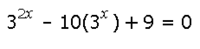# Indices Equation

#### Attachments

• 1.3 KB Views: 0

#### Algebraneophyte

##### New member
A reply to myself lol.... Solved it. x = 0 and 2.

#### Subhotosh Khan

##### Super Moderator
Staff member
Hi.

How would you find the two solutions to the following? It looks somewhat like a quadratic. I believe you can generate a dummy variable such as (3^x)= Y and (3^2x) = Y^2

View attachment 27189

Thank you for your time.
If I were to solve this problem:

I would substitute → u = 3x..............then→ u2 - 10*u + 9 = 0 ← This is a quadratic equation.

Solution of the equation is u = 1 or 9 → x = ??